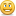# need a javascript and explanation

Hi,

I have a number that I need to convert to a integer (no decimal places) from a calculations. Perhaps I have taken the long way to do this so I am looking for helpful advise.

basically I use the following variables:

totalScore (this adds up all the results from the various variables in the questions)

totalPossible (this is set to 285)

totalPercent (the result of the calculation)

I set totalPercent to totalScore on load

divide totalPercent by totalPossible

this results in as example 28.54

what I need is to round 28.54 to an integer (29)

I think my javascript is off so if anyone can provide an example I would be so appreciativevar player=GetPlayer();
var totalPercent=player.GetVar("totalPercent");
var mathround=Math.round("totalPercent");